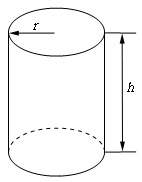Paul's Online Notes
Home / Calculus I / Applications of Derivatives / Optimization
Show Mobile Notice Show All Notes Hide All Notes
Mobile Notice
You appear to be on a device with a "narrow" screen width (i.e. you are probably on a mobile phone). Due to the nature of the mathematics on this site it is best views in landscape mode. If your device is not in landscape mode many of the equations will run off the side of your device (should be able to scroll to see them) and some of the menu items will be cut off due to the narrow screen width.

### Section 4.8 : Optimization

7. We want to construct a cylindrical can with a bottom but no top that will have a volume of 30 cm3. Determine the dimensions of the can that will minimize the amount of material needed to construct the can.

Show All Steps Hide All Steps

Start Solution

The first step is to do a quick sketch of the problem. We could probably skip the sketch in this case, but that is a really bad habit to get into. For many of these problems a sketch is really convenient and it can be used to help us keep track of some of the important information in the problem and to “define” variables for the problem.

Here is the sketch for this problem.Show Step 2

Next, we need to set up the constraint and equation that we are being asked to optimize.

We are told that the volume of the can must be 30 cm3 and so this is the constraint.

$30 = \pi {r^2}h$ We are being asked to minimize the amount of material needed to construct the can,

$A = 2\pi rh + \pi {r^2}$

Recall that the can will have no top and so the second term will only be for the area of the bottom of the can.

Show Step 3

Now, le solve the constraint for $$h$$ (that will allow us to avoid dealing with roots).

$h = \frac{{30}}{{\pi {r^2}}}$

Plugging this into the amount of material function gives,

$A\left( r \right) = 2\pi r\left( {\frac{{30}}{{\pi {r^2}}}} \right) + \pi {r^2} = \frac{{60}}{r} + \pi {r^2}$ Show Step 4

Finding the critical point(s) for this shouldn’t be too difficult at this point. Here is the derivative.

$A'\left( r \right) = - \frac{{60}}{{{r^2}}} + 2\pi r = \frac{{2\pi {r^3} - 60}}{{{r^2}}}$

From this it looks like the only critical point is : $$r = \sqrt{\frac{60}{2\pi}} = 2.1216$$.

Note that $$r=0$$ can’t be a critical point because the function does not exist there.

Be careful here and do not get into the habit of just eliminating the zero as a critical point. The only reason for eliminating it in this case is for physical reasons. If we had just given the equations without any physical reasoning it would have to be included in the rest of the work!

Show Step 5

The second derivative of the volume function is,

$A''\left( r \right) = \frac{{120}}{{{r^3}}} + 2\pi$

From this we can see that the second derivative is always positive for positive $$r$$ (which we will always have for this case since $$r$$ is the radius of a can). Therefore, provided $$r$$ is positive, $$A\left( r \right)$$ will always be concave up and so the single critical point we got in Step 4 must be a relative minimum and hence must be the value that gives a minimum amount of material.

Show Step 6

Now, let’s finish the problem by getting the height of the can.

$h = \frac{{30}}{{\pi {{\left( {2.1216} \right)}^2}}} = 2.1215$

The final dimensions are then,

$\require{bbox} \bbox[2pt,border:1px solid black]{{r = 2.1216\hspace{1.0in}h = 2.1215}}$